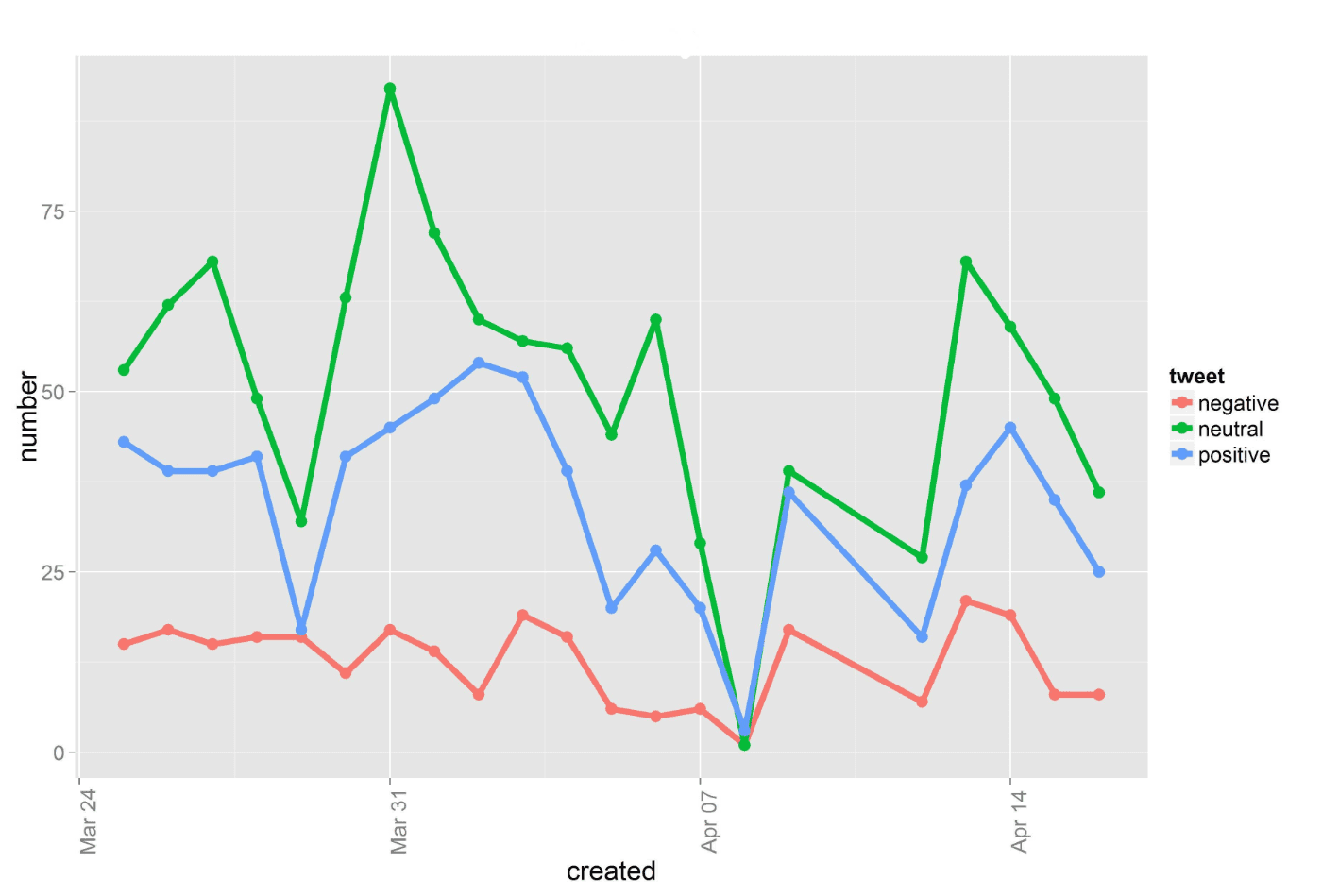Recently I’ve designed a relatively simple code in R for analyzing Twitter posts content via calculating the number of positive, negative and neutral words. The idea of processing tweets is based on the presentation http://www.slideshare.net/ajayohri/twitter-analysis-by-kaify-rais.

The words in the tweet correspond with the words in dictionaries that you can find on the internet or create by yourself. It is also possible to edit these dictionaries. Really great work, but I’ve discovered some issue.

There are some limitations in the API of Twitter. It depends on the total number of tweets you access via API but usually, you can get tweets for the last 7-8 days (not longer, and it can be 1-2 days only). The 7 to 8 days time limit doesn’t allow us to analyze historical trends.

My idea is to create a storage file in order to accumulate historical data and bypass API’s limitations. If you extract tweets regularly, you would analyze the dynamics of sentiments with the chart like this one:Furthermore, this algorithm includes a function that allows you to extract quite a few keywords that you are interested in. The process can be repeated several times a day and data set for each keyword will be saved separately. It can be helpful, for example, for doing competitors analysis.

```#connect all libraries
library(ROAuth)
library(plyr)
library(dplyr)
library(stringr)
library(ggplot2)```
```#connect to API
consumerKey <- '____________' #put the Consumer Key from Twitter Application
consumerSecret <- '______________'  #put the Consumer Secret from Twitter Application
Cred <- OAuthFactory\$new(consumerKey=consumerKey,
consumerSecret=consumerSecret,
requestURL=reqURL,
accessURL=accessURL,
authURL=authURL)
Cred\$handshake(cainfo = system.file('CurlSSL', 'cacert.pem', package = 'RCurl')) #There is URL in Console. You need to go to, get code and enter it in Console```
```save(Cred, file='twitter authentication.Rdata')
load('twitter authentication.Rdata') #Once you launched the code first time, you can start from this line in the future (libraries should be connected)
```#the function for extracting and analyzing tweets
search <- function(searchterm)
{
#extact tweets and create storage file```
```list <- searchTwitter(searchterm, cainfo='cacert.pem', n=1500)
df <- twListToDF(list)
df <- df[, order(names(df))]
df\$created <- strftime(df\$created, '%Y-%m-%d')
if (file.exists(paste(searchterm, '_stack.csv'))==FALSE) write.csv(df, file=paste(searchterm, '_stack.csv'), row.names=F)```
```#merge the last extraction with storage file and remove duplicates
stack <- rbind(stack, df)
stack <- subset(stack, !duplicated(stack\$text))
write.csv(stack, file=paste(searchterm, '_stack.csv'), row.names=F)```
```#tweets evaluation function
score.sentiment <- function(sentences, pos.words, neg.words, .progress='none')
{
require(plyr)
require(stringr)
scores <- laply(sentences, function(sentence, pos.words, neg.words){
sentence <- gsub('[[:punct:]]', "", sentence)
sentence <- gsub('[[:cntrl:]]', "", sentence)
sentence <- gsub('\\d+', "", sentence)
sentence <- tolower(sentence)
word.list <- str_split(sentence, '\\s+')
words <- unlist(word.list)
pos.matches <- match(words, pos.words)
neg.matches <- match(words, neg.words)
pos.matches <- !is.na(pos.matches)
neg.matches <- !is.na(neg.matches)
score <- sum(pos.matches) - sum(neg.matches)
return(score)
}, pos.words, neg.words, .progress=.progress)
scores.df <- data.frame(score=scores, text=sentences)
return(scores.df)
}```
```pos <- scan('C:/___________/positive-words.txt', what='character', comment.char=';') #folder with positive dictionary
neg <- scan('C:/___________/negative-words.txt', what='character', comment.char=';') #folder with negative dictionary
neg.words <- c(neg, 'wtf', 'wait', 'waiting', 'epicfail')```
```Dataset <- stack
Dataset\$text <- as.factor(Dataset\$text)
scores <- score.sentiment(Dataset\$text, pos.words, neg.words, .progress='text')
write.csv(scores, file=paste(searchterm, '_scores.csv'), row.names=TRUE) #save evaluation results```
```#total score calculation: positive / negative / neutral
stat <- scores
stat\$created <- stack\$created
stat\$created <- as.Date(stat\$created)
stat <- mutate(stat, tweet=ifelse(stat\$score > 0, 'positive', ifelse(stat\$score < 0, 'negative', 'neutral')))
by.tweet <- group_by(stat, tweet, created)
by.tweet <- summarise(by.tweet, number=n())
write.csv(by.tweet, file=paste(searchterm, '_opin.csv'), row.names=TRUE)```
```#chart
ggplot(by.tweet, aes(created, number)) + geom_line(aes(group=tweet, color=tweet), size=2) +
geom_point(aes(group=tweet, color=tweet), size=4) +
theme(text = element_text(size=18), axis.text.x = element_text(angle=90, vjust=1)) +
#stat_summary(fun.y = 'sum', fun.ymin='sum', fun.ymax='sum', colour = 'yellow', size=2, geom = 'line') +
ggtitle(searchterm)```
`ggsave(file=paste(searchterm, '_plot.jpeg'))`
`}`
`search("______") #enter keyword`

Finally, we will get four files:

• storage file with initial data,
• file with tweets rating,
• file with the number of tweets of each type (positive / negative / neutral) as of date,
• and the chart that looks like:SaveSave